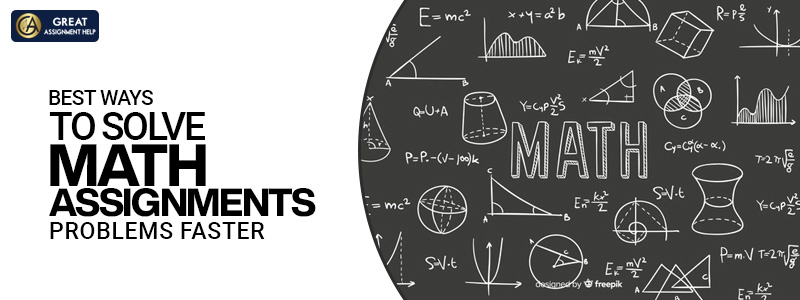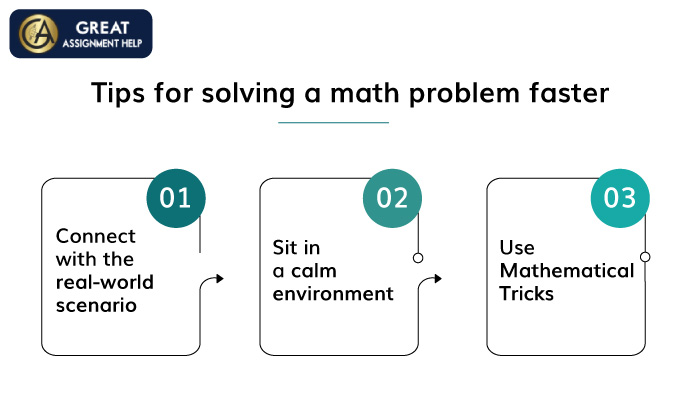4.9/5

5 Star Rating

93940

Orders Deliver

3949

PhD Experts

24x7

Support

100%

Privacy

100%

Top Quality

# Best ways to solve Math Assignments Problems faster

A subject which has high hatred among a majority of the students is ‘Mathematics’. Solve a math assignments problem itself is a big problem for many. No matter whether you love or hate Maths, it is mandatory to learn the subject because the basic calculations with numbers have a high level of importance in our daily life. Also, it will help you gain problem-solving skills.

When it comes to “Mathematics”, finding the desired solution for a problem is not at all an easy task. No matter how well you prepare, at times you will end up getting low scores in the exams. For getting good grades, you must be aware of how to crack a numerical problem faster with the desired strategies and techniques.

Ways to solve Math Assignments

Tips for solving a math problem faster

Conclusion## Ways to solve Math Assignments

Follow the best possible ways mentioned below to solve the online Maths Assignment faster.

### Understand the concept

Memorizing a math problem is one common mistake that is being committed by students. If you wish to score good marks in maths, then never memorize a problem or a process. Memorizing is one of the bad ways of learning.

To solve math problems quickly, you must know the logic and the concept behind it. If you understand the entire process of solving a problem and implement them step by step, then ultimately it will be easy for you to find the answers. Also, you may start liking the subject if you are clear with the concepts.

### Keep practicing

You can’t taste success without hard work. To become a pro at solving mathematical problems, you should work out daily.

If you practice regularly, then automatically your problem-solving skills will be fine-tuned, and you will also end up solving the problems quickly. So, spend your free time solving mathematical problems and then observe the change in you.

### Check for errors

There will be multiple approaches or methods to solve a problem. Once you find the answer, check the entire solution step by step once or twice to make sure whether your answer is right or wrong. Reviewing your answers will help to spot your mistakes and rectify them. Also, you can strengthen your mathematical skills and avoid doing the same mistakes repeatedly you review a problem.

## Tips for solving a math problem faster

To develop your math skills and to solve a problem faster, follow the below-mentioned tips.### Connect with the real-world scenario

It is usually difficult to understand mathematical problems. So, to make it easy to remember and understand, connect the problem and its solutions with a real-world scenario. If you compare it with real-world examples, then you would get a better idea while solving. The topics like basic arithmetic, probability, distance, metrics, etc. will be more relatable to our day-to-day life.

### Sit in a calm environment

To solve a math problem, high concentration is required. If you sit in a noisy environment, then you may not understand the problem properly or write wrong numbers while doing calculations. So, it is always better to isolate yourself and sit in an environment that is free from distractions to solve problems faster and get the correct results.

### Use Mathematical Tricks

There are many tricks and shortcuts available for doing multiplication, addition, subtraction, division, and calculating percentage. So, learn all the quick and easy calculation tricks to solve a problem quickly.

## Conclusion

Solve a math assignments problem requires a lot of skills and practice. You can’t become experts over the night. So, keep practicing to solve the problems faster. If you follow all the important tips and ways mentioned in this post, then you may excel in mathematics. For solving difficult calculations, use a calculator. Also, research and get to know about the different techniques and easy strategies to solve a math problem quickly. Still confused about how to solve critical math problems connect Math Homework helpers.